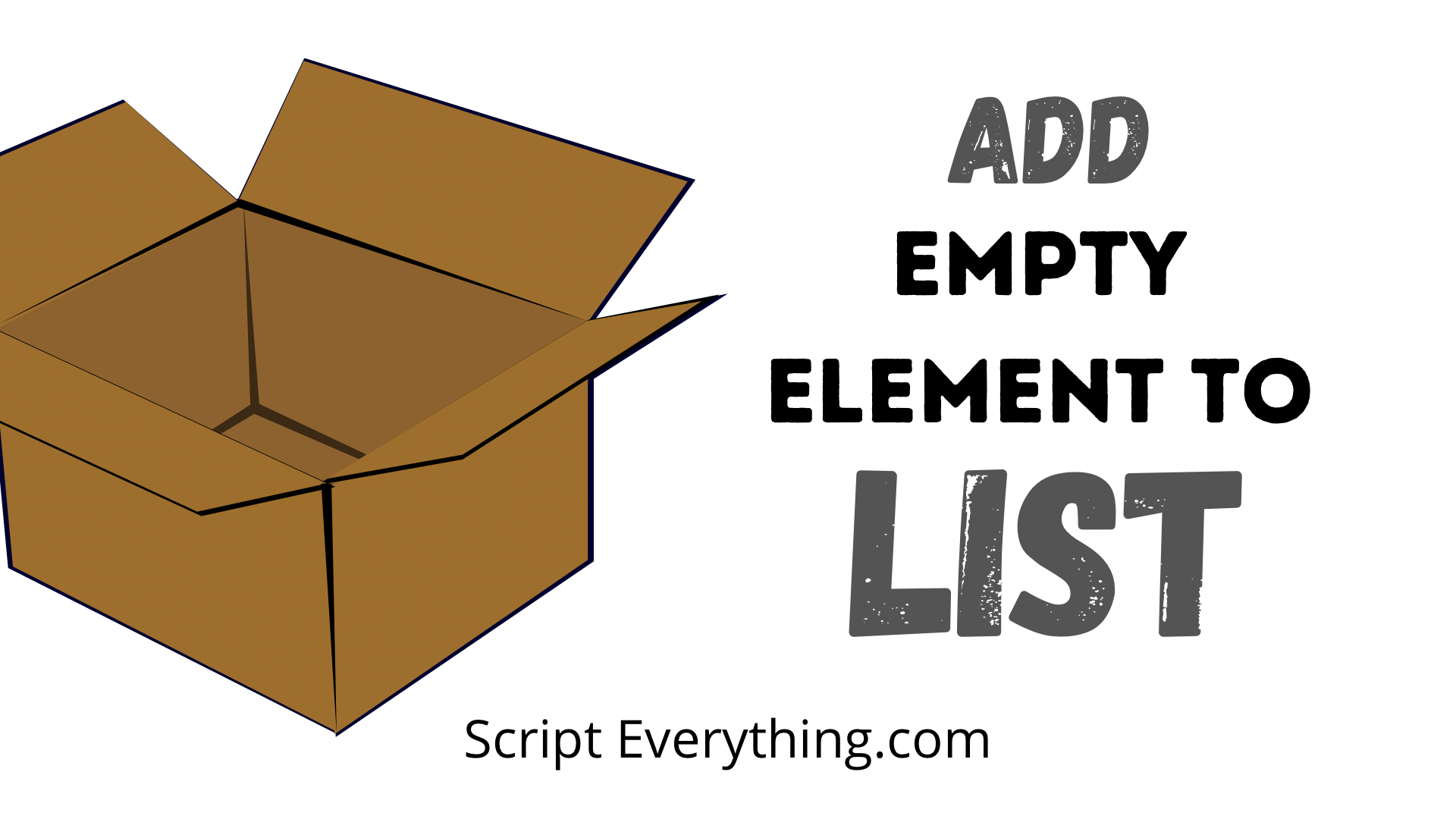# How To Add An Empty Element To A List In PythonTo insert or append an empty element into a Python list you need to first define what is meant by the word empty . By empty do you mean an empty string, ``` None ``` or something else? While I may use ``` None ``` in this post you can easily substitute it for something else according to your requirements.

To add an empty element into a list either replace the existing element with the empty variable or if inserting it at the end use the list method ``` .append() ``` or if inserting it at a specific place use the other list method ``` .insert() ``` .

Here are some examples demonstrating these techniques:

``````>>> e = [1, 2, 3]
>>> e = None
>>> print(e)
[None, 2, 3]``````

In this first example, you can add your empty element into the list by simply referencing the index of the list item you want to change to an empty element and by using the assignment character ``` = ``` setting that element to ``` None ``` .

``````>>> e.append(None)
>>> print(e)
[None, 2, 3, None]``````

To add an empty element automatically to the end of the list simply use the ``` .append() ``` list method which has a single parameter that can be of any data type.

``````>>> e.insert(2, None}
>>> print(e)
[None, 2, None, 3, None]``````

Another helpful list method to insert an empty element anywhere within the list is to use ``` .insert(i, x) ``` which has two parameters: the first being the index number where the new element will be added into the last and the second parameter being the item to insert.

As can be seen from the example above, the first parameter was ``` 2 ``` which with the original list contained the element ``` 3 ``` this was where the empty element was added and everything on and after that element was pushed back.

## How To Start A List With Empty Elements

What if you want to start a list already prepopulated with empty items? How can you achieve this in Python?

Thankfully in Python, it is relatively easy to get started with a list already populated with empty items and this can be achieved in one line of code. Suppose I needed to start a list with 5 empty elements, here’s how this would work:

``````>>> e = [None] * 5
>>> print(e)
[None, None, None, None, None]``````

By using the multiplication operator on the list literal which contained one empty element Python that uses this and applies it 5 times creating a list with 5 empty elements.

## How To Add Empty Elements By Condition

What if you needed to replace the elements in a list based on a condition? Can this be done in Python?

Again Python makes it relatively straightforward in being able to modify elements within a list according to certain criteria, and what’s even more impressive is that this can be done on one line of code!

The example used below will demonstrate how you can change each of the elements below in the list where the number exceeds an amount:

``````>>> e = [101, 99, 103, 95]
>>> f = [elem if elem > 100 else None for elem in e]
>>> print(f)
[101, None, 103, None]``````

In the second line of code a list comprehension is used to create a new list. Starting at the end of the list comprehension code with the for loop the code automatically loops through each element of the list ``` e ``` and assigns each element with the variable ``` elem ``` .

With each iteration of the for loop the one line if condition is triggered where each element is checked against the condition used, being in this case if the ``` elem ``` is greater than the value of 100. If this condition is true the statement made at the start of the ``` if ``` condition is used, being in this case just returning ``` elem ``` , however if this condition is not true for the element then ``` None ``` is used.

By using a list comprehension along with an ``` if ``` condition on each element you can replace elements in your list to empty with one line of code.

## Summary

To add an empty element into a Python list use either a direct index reference to the list item that needs replacing or use the list method ``` .append(None) ``` to insert the empty element at the end of the list or use the other list method ``` .insert(idx, None) ``` to insert the empty element at a specific point in the list.

Next, you might want to find out how to check if your list is empty .

Author of scripteverything.com, Ryan has been dabbling in code since the late '90s when he cut his teeth by exploring VBA in Excel when trying to do something more. Having his eyes opened with the potential of automating repetitive tasks, he expanded to Python and then moved over to scripting languages such as HTML, CSS, Javascript and PHP. When he is not behind a screen, Ryan enjoys a good bush walk with the family during the cooler months, and going with them to the beach during the warmer months.